# Pytorch入门教程01-张量(Tensor)的介绍2020年10月1日07:49:35

3 9858字阅读32分51秒

## 简介

PyTorch 中的所有操作都是在张量的基础上进行的。作为 Pytorch 的一份入门的资料，我们就会首先从张量开始讲起，主要分为下面几个部分：

• 张量的定义；
• 张量的运算；
• 张量的切片；
• 改变张量形状；
• GPU 还是 CPU；

1. import torch

## 张量的简单介绍

PyTorch 中的所有内容都基于 Tensor 操作。我们可以使用 empty 来创建一维、二维、或是三维的张量。在这里我们会介绍一下几种初始化张量的方式：

• 一维张量（Scalars），利用 item 转换为 python float 类型
• 张量的创建
• 创建空张量, torch.empty.
• 创建随机张量, torch.rand或torch.randn.
• 创建全0或全1张量, torch.zeros和torch.ones
• 创建指定值的张量, torch.tensor(List)
• Numpy与Tensor的相互转化(比较常用)
• 张量的类型, 使用 dtype 查看或指定张量的类型
• 张量是否自动计算梯度, `requires_grad=True`进行指定.

### 一维张量（Scalars）

1. x = torch.tensor([3.0])
2. y = torch.tensor([2.0])
3. print('x + y = ', x + y)
4. print('x * y = ', x * y)
5. print('x / y = ', x / y)
6. print('x ** y = ', torch.pow(x,y))
7. """
8. x + y =  tensor([5.])
9. x * y =  tensor([6.])
10. x / y =  tensor([1.5000])
11. x ** y =  tensor([9.])
12. """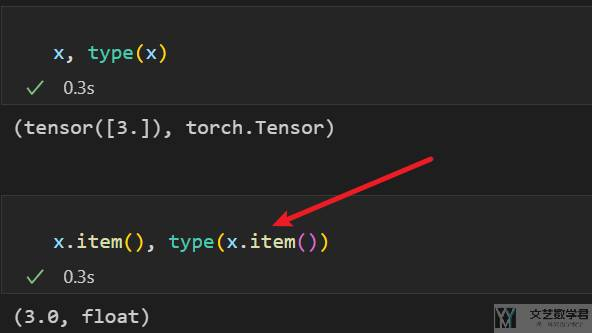### 张量的创建

`torch.empty`可以初始化指定大小的张量, 如果不指定值的话, 内容为随机值. (注意, 我们可以通过`x.size`查看张量的大小)

1. x = torch.empty(2)
2. print(x.size())
3. # >>  torch.Size()
4. x = torch.empty(2,3)
5. print(x.size())
6. # >> torch.Size([2, 3])
7. x = torch.empty(1,2,3)
8. print(x.size())
9. # >>  torch.Size([1, 2, 3])

1. torch.rand(2,3)
2. """
3. tensor([[0.1180, 0.6572, 0.6190],
4.         [0.9897, 0.8449, 0.1371]])
5. """

1. x = torch.zeros(2, 2) # 全0张量
2. print(x)
3. """
4. tensor([[0., 0.],
5.         [0., 0.]])
6. """
7. y = torch.ones(2, 2) # 全1张量
8. print(y)
9. """
10. tensor([[1., 1.],
11.         [1., 1.]])
12. """

1. x = torch.tensor([[1,2],[3,4]])
2. print(x)
3. """
4. tensor([[1, 2],
5.         [3, 4]])
6. """

1. # numpy -> tensor
2. x = np.ones((2,3))
3. y = torch.from_numpy(x)
4. print(y)
5. """
6. tensor([[1., 1., 1.],
7.         [1., 1., 1.]], dtype=torch.float64)
8. """
9. # tensor -> numpy
10. x = torch.zeros(2,3)
11. y = x.numpy()
12. print(type(y))
13. # <class 'numpy.ndarray'>

1. # 特征数据
2. X_train = torch.from_numpy(X_train.astype(np.float32))
3. X_test = torch.from_numpy(X_test.astype(np.float32))
4. # 标签数据
5. y_train = torch.from_numpy(y_train.astype(np.long))
6. y_test = torch.from_numpy(y_test.astype(np.long))

### 张量的类型

1. x = torch.rand(2,2)
2. print(x.dtype)
3. # torch.float32

1. x = torch.rand(2, 2, dtype=torch.float64)
2. print(x)
3. """
4. tensor([[0.0942, 0.6157],
5.         [0.6045, 0.1863]], dtype=torch.float64)
6. """
7. # check type
8. print(x.dtype)
9. # torch.float64

### 指定张量是否计算梯度

1. x = torch.tensor([[1,2],[3,4]], dtype=torch.float32, requires_grad=True)
2. print(x)
3. """
4. tensor([[1., 2.],
6. """

## 张量的运算

### 基础运算

1. x = torch.ones(2,2)
2. y = torch.ones(2,2)
3. # 加法
5. print(z)
6. """
7. tensor([[2., 2.],
8.         [2., 2.]])
9. """
10. # 减法
11. z = torch.sub(x, y)
12. print(z)
13. """
14. tensor([[0., 0.],
15.         [0., 0.]])
16. """
17. # 乘法
18. z = torch.mul(x, y)
19. print(z)
20. """
21. tensor([[1., 1.],
22.         [1., 1.]])
23. """
24. # 除法
25. z = torch.div(x, y)
26. print(z)
27. """
28. tensor([[1., 1.],
29.         [1., 1.]])
30. """

### 向量乘法-dot product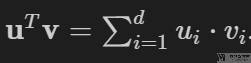1. x = torch.arange(4, dtype = torch.float32)
2. y = torch.ones(4, dtype = torch.float32)
3. print(x, y, torch.dot(x, y))
4. """
5. tensor([0., 1., 2., 3.]) tensor([1., 1., 1., 1.]) tensor(6.)
6. """

### 矩阵向量乘法-torch.mv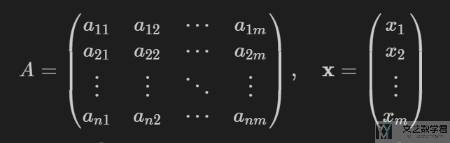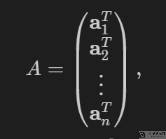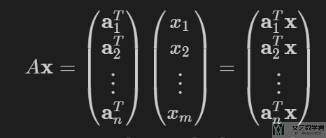1. A = torch.arange(20, dtype=torch.float32).reshape((5,4))
2. x = torch.arange(4, dtype = torch.float32)
3. torch.mv(A, x)
4. """
5. tensor([ 14.,  38.,  62.,  86., 110.])
6. """

### 矩阵与矩阵乘法-torch.mm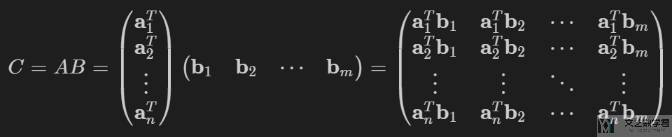1. A = torch.arange(20, dtype=torch.float32).reshape((5,4))
2. B = torch.ones(size=(4, 3))
3. torch.mm(A, B)
4. """
5. tensor([[ 6.,  6.,  6.],
6.         [22., 22., 22.],
7.         [38., 38., 38.],
8.         [54., 54., 54.],
9.         [70., 70., 70.]])
10. """

### Batch 的矩阵乘法-torch.bmm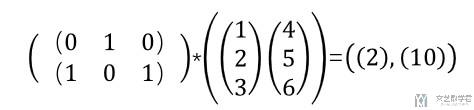1. # torch.bmm测试-这是矩阵乘法
2. x_test = torch.tensor([[[0,1,0]],[[1,0,1]]])
3. y_test = torch.tensor([[,,],[,,]])
4. print(x_test.shape,y_test.shape)
5. print(torch.bmm(x_test,y_test))
6. """
7. torch.Size([2, 1, 3]) torch.Size([2, 3, 1])
8. tensor([[[ 2]],
9.         []])
10. """

### 按行按列求和

1. X = torch.tensor([[1., 2., 3.], [4., 5., 6.]])
2. torch.sum(X, dim=1)
3. """
4. tensor([ 6., 15.])
5. """

1. X = torch.tensor([[1., 2., 3.], [4., 5., 6.]])
2. torch.sum(X, dim=1, keepdim=True)
3. """
4. tensor([[ 6.],
5.         [15.]])
6. """

### 转置运算

1. A = torch.arange(20, dtype=torch.float32).reshape((5,4))
2. print(A)
3. """
4. tensor([[ 0.,  1.,  2.,  3.],
5.         [ 4.,  5.,  6.,  7.],
6.         [ 8.,  9., 10., 11.],
7.         [12., 13., 14., 15.],
8.         [16., 17., 18., 19.]])
9. """

1. print(A.t())
2. """
3. tensor([[ 0.,  4.,  8., 12., 16.],
4.         [ 1.,  5.,  9., 13., 17.],
5.         [ 2.,  6., 10., 14., 18.],
6.         [ 3.,  7., 11., 15., 19.]])
7. """

## 张量的切片

1. x = torch.rand(2, 3)
2. print(x)
3. print(x[1, 1])  # 表示第二行, 第二列的值. (2,2)
4. print(x[:, 0])  # 第1列
5. print(x[1, :])  # 第2行
6. """
7. tensor([[0.9756, 0.6967, 0.5652],
8.         [0.1255, 0.2977, 0.6983]])
9. tensor(0.2977)
10. tensor([0.9756, 0.1255])
11. tensor([0.1255, 0.2977, 0.6983])
12. """

## 内存节省(关于Savig Memory)

• x[:] = expression
• x += y

1. z = torch.zeros_like(y)
2. print('id(z):', id(z))
3. z = x + y # 这样赋值会重新分配内存
4. print('id(z):', id(z))
5. """
6. id(z): 140029165236864
7. id(z): 140029165234768
8. """

1. z = torch.zeros_like(y)
2. print('id(z):', id(z))
3. z[:] = x + y # 这样是不会重新分配内存的
4. print('id(z):', id(z))
5. """
6. id(z): 140029165234848
7. id(z): 140029165234848
8. """

1. print(id(x))
2. print(x)
3. """
4. 140029165148272
5. tensor([[40., 38., 44., 42.],
6.         [38., 40., 42., 44.],
7.         [32., 33., 34., 35.]])
8. """
9. print(y)
10. """
11. tensor([[14., 13., 16., 15.],
12.         [13., 14., 15., 16.],
13.         [12., 12., 12., 12.]])
14. """

1. x += y
2. print(id(x))
3. print(x)
4. """
5. 140029165148272
6. tensor([[54., 51., 60., 57.],
7.         [51., 54., 57., 60.],
8.         [44., 45., 46., 47.]])
9. """

## 转换为 One-hot 向量

1. batch_size = 5
2. nb_digits = 10
3. # Dummy input that HAS to be 2D for the scatter (you can use view(-1,1) if needed)
4. y = torch.LongTensor(batch_size,1).random_() % nb_digits
5. # One hot encoding buffer that you create out of the loop and just keep reusing
6. y_onehot = torch.FloatTensor(batch_size, nb_digits)
7. # In your for loop
8. y_onehot.zero_()
9. y_onehot.scatter_(1, y, 1)
10. print(y)
11. """
12. tensor([,
13.         ,
14.         ,
15.         ,
16.         ])
17. """
18. print(y_onehot)
19. """
20. tensor([[0., 0., 1., 0., 0., 0., 0., 0., 0., 0.],
21.         [0., 1., 0., 0., 0., 0., 0., 0., 0., 0.],
22.         [0., 0., 0., 0., 0., 0., 0., 1., 0., 0.],
23.         [0., 0., 0., 0., 1., 0., 0., 0., 0., 0.],
24.         [0., 0., 0., 0., 0., 0., 0., 0., 0., 1.]])
25. """

## 改变张量形状

### 使用 view 或是 reshape 修改形状

1. x = torch.randn(4, 4)
2. y = x.view(1, 16)  # 指定改变后的大小
3. z = x.view(2, 8)
4. print(x.size(), y.size(), z.size())
5. # torch.Size([4, 4]) torch.Size([1, 16]) torch.Size([2, 8])

1. x = torch.randn(4, 4)
2. y = x.reshape(1, 16)  # 指定改变后的大小
3. z = x.reshape(2, 8)
4. print(x.size(), y.size(), z.size())
5. # torch.Size([4, 4]) torch.Size([1, 16]) torch.Size([2, 8])

1. x = torch.ones(3,5,5)
2. y = x.view(3,-1)
3. print(y.size())
4. # torch.Size([3, 25])

### 使用 squeeze 和 unsqueeze 添加或删除维度

`squeeze``unsqueeze` 是两个非常实用的功能，用来添加或是删除维度。下面是官方的定义：

• The `squeeze` method "returns a tensor with all the dimensions of `input` of size 1 removed",
• The`unsqueeze` "returns a new tensor with a dimension of size one inserted at the specified position".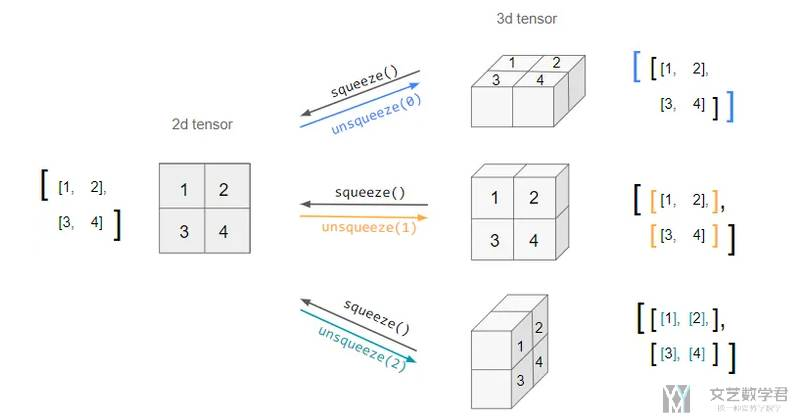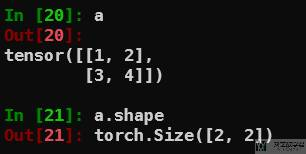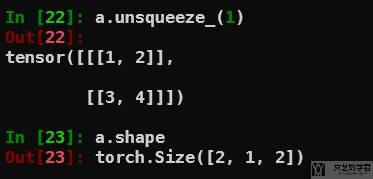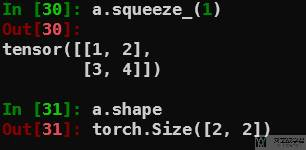### 使用 expand 重复 tensor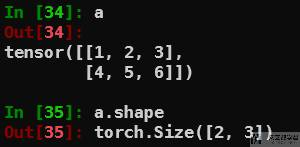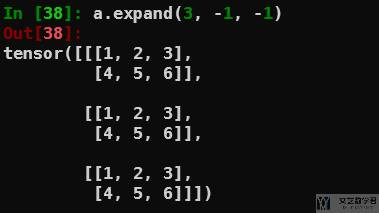### 使用 transpose 交换 axis（swap the axis）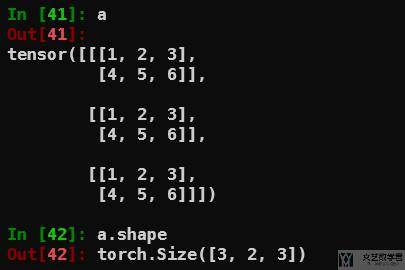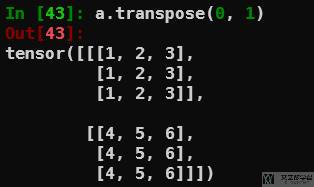### 使用 permute 交换任意多个 axis（swap the axis）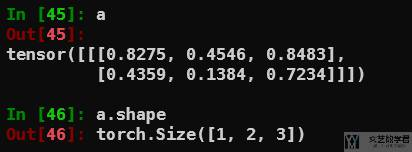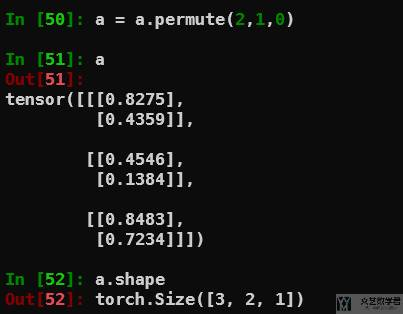## 广播机制

1. x = torch.arange(1, 4).view(3, 1)
2. print(x)
3. """
4. tensor([,
5.         ,
6.         ])
7. """
8. y = torch.arange(4, 6).view(1, 2)
9. print(y)
10. """
11. tensor([[4, 5]])
12. """

1. print(x + y)
2. """
3. tensor([[5, 6],
4.         [6, 7],
5.         [7, 8]])
6. """

## GPU 还是 CPU

1. torch.cuda.is_available()

1. device = torch.device('cuda' if torch.cuda.is_available() else 'cpu')
2. x = torch.tensor([1,2,3]).to(device)

• 微信公众号
• 关注微信公众号
•• QQ群
• 我们的QQ群号
•• 本文由 发表于 2020年10月1日07:49:35
• 转载请务必保留本文链接：https://mathpretty.com/12493.html